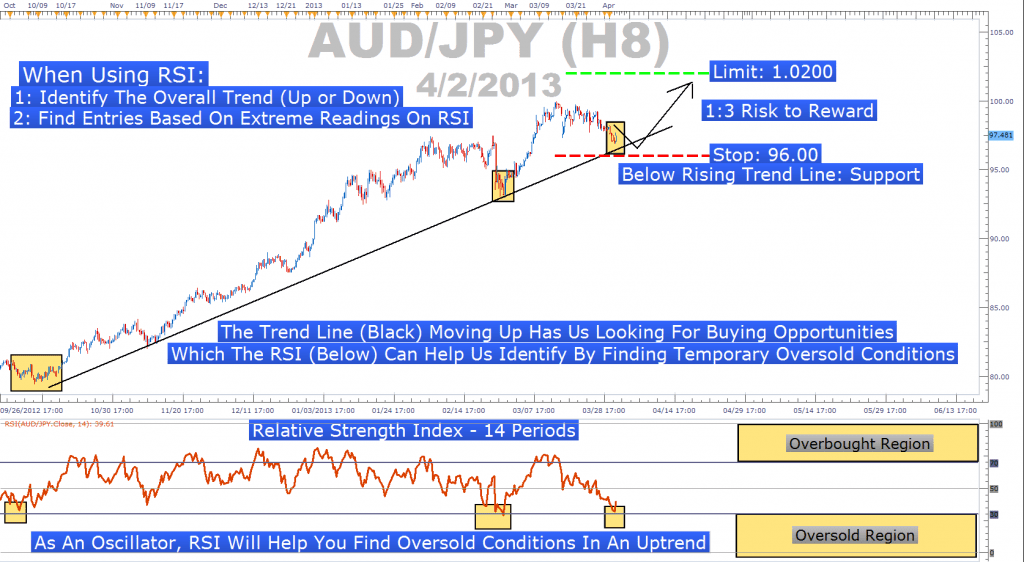Forex RSI Indicator – formula calculation and RSI strategy

Relative Strength Index or the RSI sign is the most popular part of the “Oscillator” breed of technical indicators. RSI was created by J. Welles Wilder, to calculate relative changes which take place in between the high and low closing prices. The Traders use this Entry and exit point at the Forex market places.
The RSI is categorized as an Oscillator, as the resultant curve varies when the values are at 0 and 100. The lines of the RSI are normally drawn at both the values of 30 and 70 as a caution signal. Any value which exceeds 85 is regarded as a selling signal or a strong overbought situation, and suppose the curve drops below 15, it is a buying signal or a strong over sold situation generates.
Formula of RSI
The Metatrader4 Trading platform typically makes use of this RSI analysis, and the formula calculation series comprises of the simple steps:
1. Select a predefined period of “X” let the standard value be “14”, even though value 8 or 9 has a tendency to be more responsive.
2. Compute Relative strength = Average of “X” periods up closes/Averages of “x” periods down closes
3. Relative Strength Index = 100- (100/ (1 + Relative strength))
The RSI is a collection of a single varying curve. The traders will at time append an exponential moving average in Red to develop the value of the trading signs. The Blue line in the chart is the RSI; the Red line denotes “EMA” for the period “8” variable. The RSI is considered as a major indicator, as its signal anticipate for a possible changing trend. The demerit is however; the fact that the timing is not essentially an outcome of the RSI, the cause for this is that the lagging moving average is to verify the RSI signals.
Large flow in the price change can be the reason for the RSI to produce untrue signals. It is evident that the RSI with other indicator will be effective. Wilder had a strong belief that the indicator was open when its values deviate from the present prices in the market place.

RSI strategy :
Dailyfx team made excellent RSI image. here you can see RSI strategy using oversold / overbought trendline strategy :